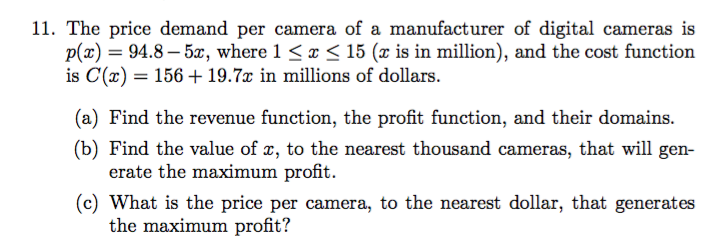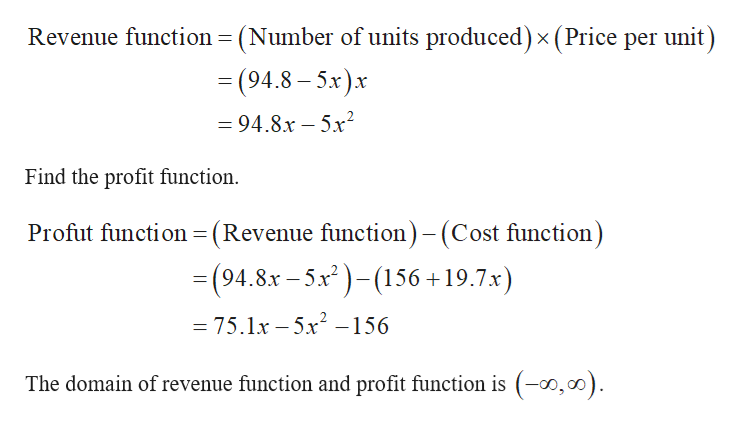# 11. The price demand per camera of a manufacturer of digital cameras isp(x)94.8-5, where 1 < x < 15 (x is in million), and the cost functionis C(a) 156 19.7x in millions of dollars(a) Find the revenue function, the profit function, and their domains(b) Find the value of x, to the nearest thousand cameras, that will generate the maximum profit(c) What is the price per camera, to the nearest dollar, that generatesthe maximum profit?

Questionhelp_outlineImage Transcriptionclose11. The price demand per camera of a manufacturer of digital cameras is p(x)94.8-5, where 1 < x < 15 (x is in million), and the cost function is C(a) 156 19.7x in millions of dollars (a) Find the revenue function, the profit function, and their domains (b) Find the value of x, to the nearest thousand cameras, that will gen erate the maximum profit (c) What is the price per camera, to the nearest dollar, that generates the maximum profit? fullscreen
check_circle

Step 1

The price demand function is given by,

Step 2

(a) Find the revenu...help_outlineImage Transcriptionclose(Number of units produced) x (Price per unit) Revenue function = (94.8-5x)x =94.8x-5x2 Find the profit function Profut function (Revenue function)- (Cost function) = (94.8x-5x)156 +19.7x) _ = 75.1x -5x -156 The domain of revenue function and profit function is (-o,00) fullscreen

### Want to see the full answer?

See Solution

#### Want to see this answer and more?

Solutions are written by subject experts who are available 24/7. Questions are typically answered within 1 hour.*

See Solution
*Response times may vary by subject and question.
Tagged in

### Calculus